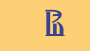Раскрыть

ISSN 2587-814X (print),
ISSN 2587-8158 (online)

Russian version: ISSN 1998-0663 (print),
ISSN 2587-8166 (online)

## Exact time-efficient combined algorithm for solving the asymmetric traveling salesman problem

2018. No. 3(45). P. 20–28 [issue contents]

For practical, important tasks in the fields of economics and logistics, as well as in a number of technical applications, it becomes necessary to solve the traveling salesman problem (TSP). Quite often, the features of these problems lead to the traveling salesman problem in asymmetric formulation (asymmetric traveling salesman problem, ATSP). Moreover, in some practical applications it is desirable to obtain an exact solution. One of the known exact algorithms for solving the ATSP is an algorithm that implements the well-known branch and bound method. The known experimental estimates of its complexity on the average are exponential. However, this does not mean that for small dimensions of the problem (currently, no more than 70–75), the expected time for solving the individual problem is unacceptably high. The need to reduce the time for solving individual problems dictated by practice is associated with the use of various modifications of this algorithm, of which a modification that involves storing truncated matrices in the search decision tree is one of the most effective. In this article, the authors rely on this modification. Other possible improvements in the time efficiency of the software implementation of the branch and bound method are related, among other things, to obtaining the initial approximation by heuristic algorithms. As a result, we get a combined algorithm, in which, at the first stage, some heuristics works to obtain the initial solution, from which the branch and bound method starts. This idea has been discussed for a long time, but the problem is that to reduce time, such a heuristic algorithm is needed that delivers a solution close to optimal which will be found quite fast. One of the possible solutions to this problem is the subject of this article.
The subject of the research in this article is the choice of the best heuristic algorithm which, when applied, leads to an increase in temporal efficiency in combination with the algorithm of the branch and bound method, and an experimental study of its software implementation in order to obtain an average time for solving individual problems. On the basis of the results obtained, recommendations are given on the limiting dimensions of the problem that allow for an acceptable solution time, something which is of interest in the practical application of this combined algorithm in the tasks of business informatics and logistics.

Citation:

Zhukova G.N., Ulyanov M.V., Fomichev M.I. (2018) Exact time-efficient combined algorithm for solving the asymmetric traveling salesman problem. BusinessInformatics, no. 3 (45), pp. 20–28. DOI: 10.17323/1998-0663.2018.3.20.28.

BiBTeX
RIS